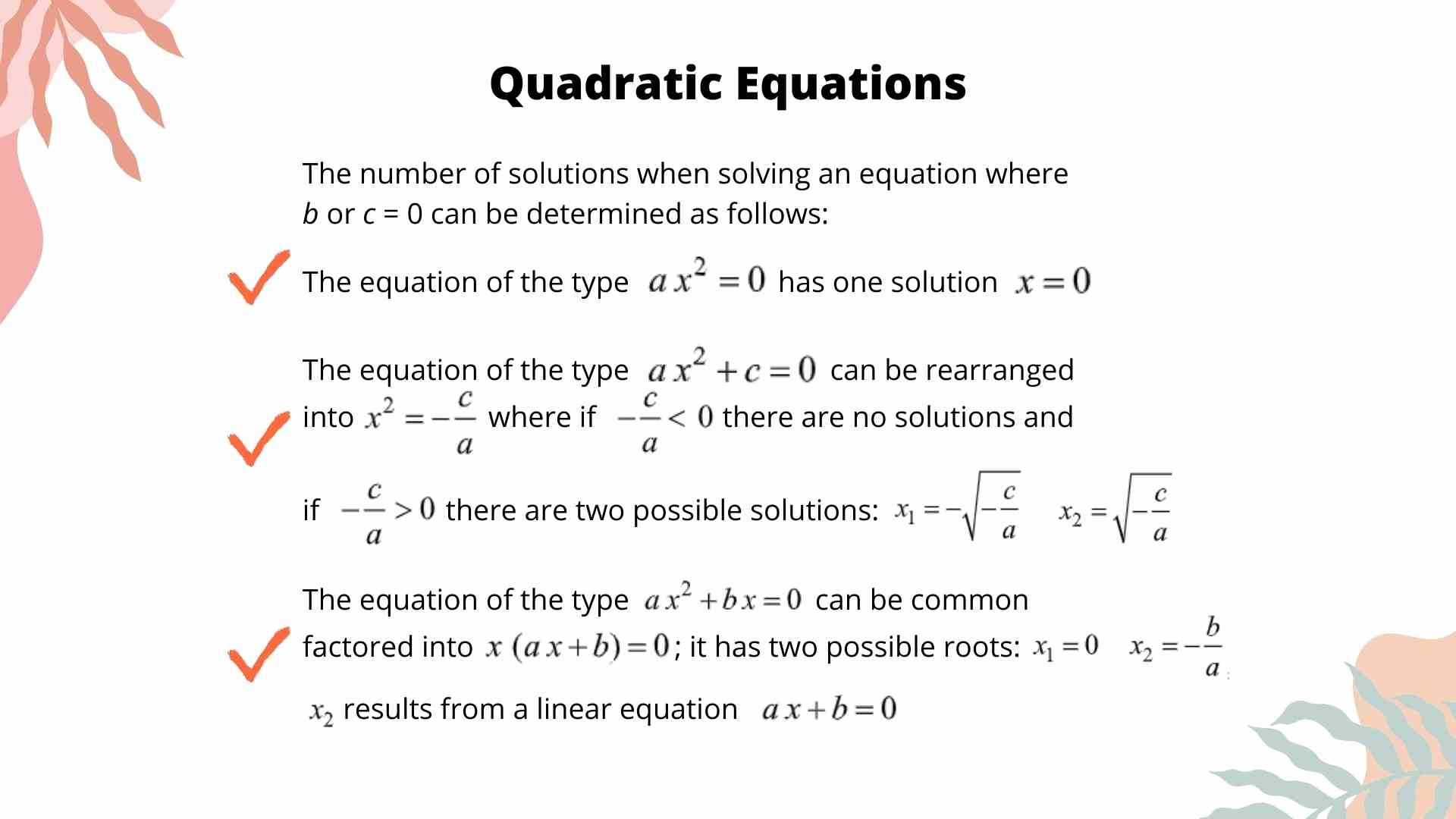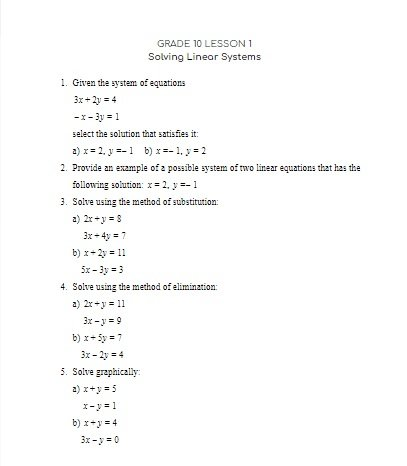CA\$2.00

Solving quadratic equations requires a good understanding of and proficiency with a number of concepts. A quadratic equation is an equation of the form ax2 + bx + c = 0. This is a standard form equation. A quadratic equation can also be recorded in the factored form a(x – r)(x – s) = 0, where r and s are the roots of the equation.

In order to determine the solutions (roots or x-intercepts of the parabola) of the quadratic equation and when the equation is given in a standard form, we can either try to factor the equation or use the Quadratic Formula.

The method of solving quadratic equations by factoring depends whether the standard form equation is given as a simple or as a complex trinomial and whether the factors could be easily identified.

If the a value of the equation is a = 1 and the equation is x2 + bx + c = 0, then x1 + x2 = -b and (x1)(x2) = c

However, if a is not 0 or 1, then the equation could be solved by factoring using the decomposition method (watch the video lesson to see how).

Sometimes factors are not very “nice” numbers. In such cases it is difficult to factor and the Quadratic Formula should be used instead.